# The line of action of force F passes through point B. Find the moment due to force F about axis AC, given:   F̄ = <1...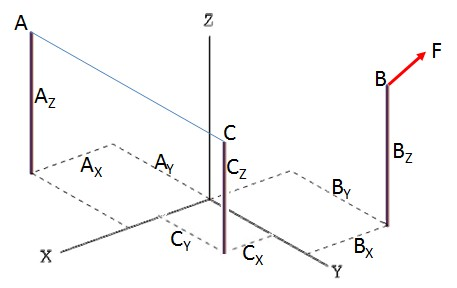The line of action of force F passes through point B. Find the moment due to force F about axis AC, given:
= <10, 70, -10> lbs,   AX = 6 ft,   AY = 3 ft,   AZ = 7 ft,   BX = 7 ft,   BY= 5 ft,   BZ = 9 ft,   CX = 5 ft,   CY = 5 ft,   CZ = 1 ft

MAC =      lb·ft

AC= <   ,   ,   >  lb·ft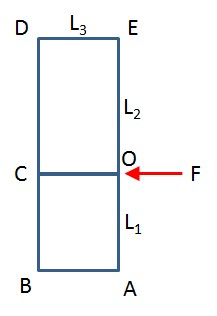Find the moment about points A, B, C, D, and E, due to force F, given:

F = 50 lbs,   L1 = 6 ft,   L2 = 7 ft,   L3 = 4 ft

MA =   lb·ft
MB =   lb·ft
MC =   lb·ft
MD =   lb·ft
ME =   lb·ft

3 ーF 1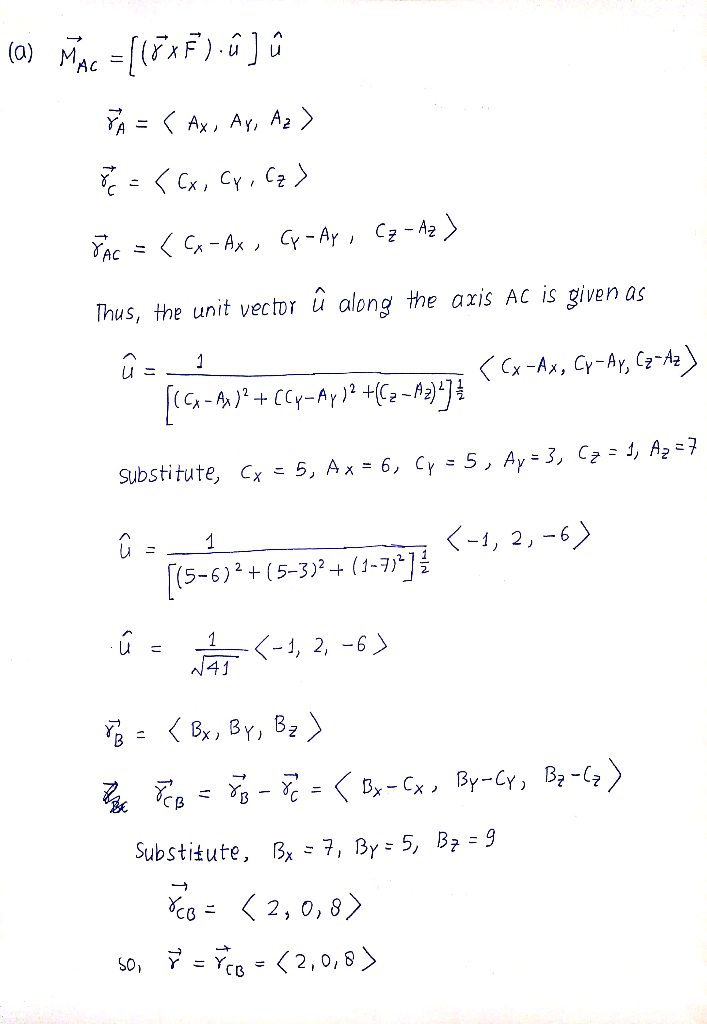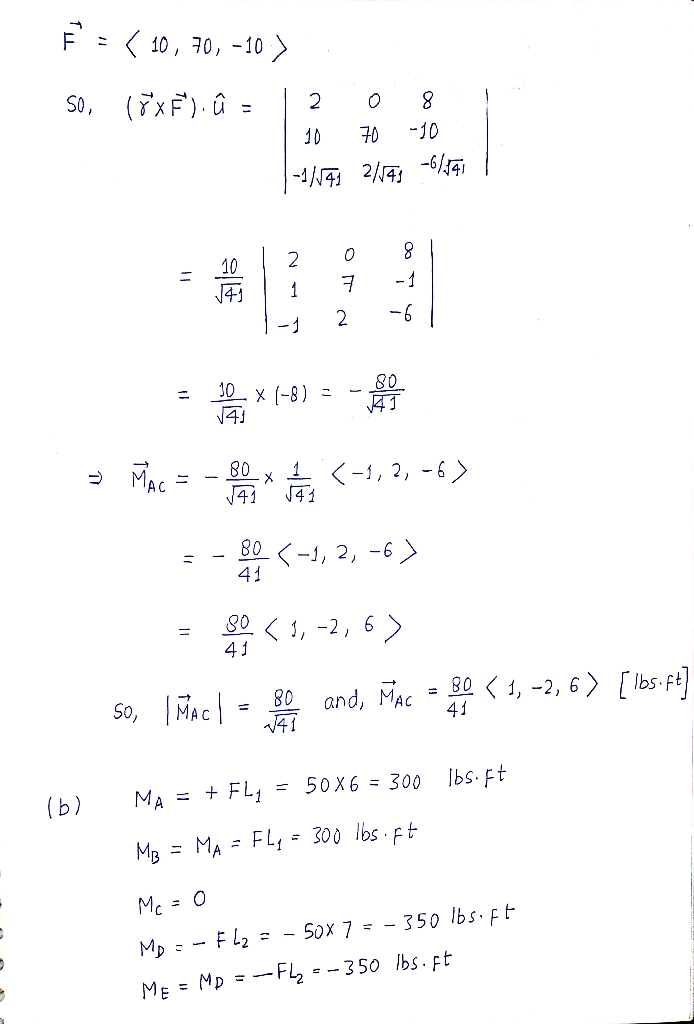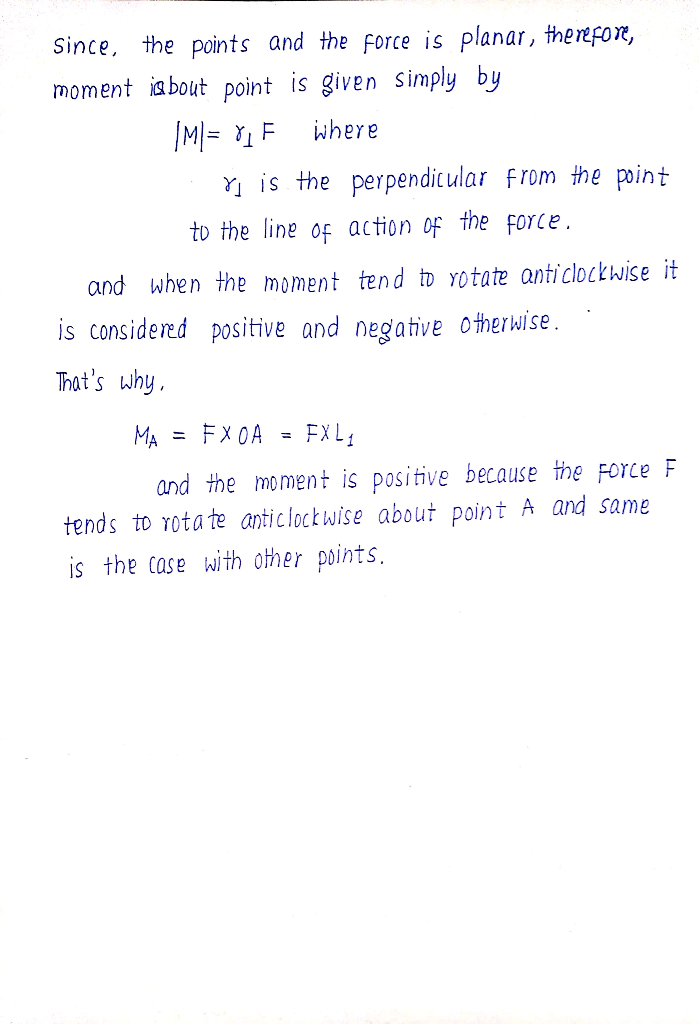##### Add Answer of: The line of action of force F passes through point B. Find the moment due to force F about axis AC, given:   F̄ = <1...
More Homework Help Questions Additional questions in this topic.